# 0708-1300/Class notes for Tuesday, November 27

Announcements go here

## Today's Agenda

• The planimeter with a picture from http://whistleralley.com/planimeter/planimeter.htm but our very own plane geometry and Stokes' theorem.
• Completion of the proof of Stokes' theorem.
• Completion of the discussion of the two- and three-dimensional cases of Stokes' theorem.
• With luck, a discussion of de-Rham cohomology, homotopy invariance and Poincaré's lemma.

## Class Notes

The notes below are by the students and for the students. Hopefully they are useful, but they come with no guarantee of any kind.

### First Hour

Planimeter

A planimeter consists of two rods connected with a join where the end of one rod is fixed (but free to rotate) and the opposing end of the second rod traces out the boundary of some surface on the plane. I.e., the planimeter is kind of like a 1 legged roach. At the join of the two rods is a wheel which rotates (and measures the rotation) when the rod tracing the boundary moves in the normal direction and simply slides back an forth when moved in a tangential direction.

Now we recall from plane geometry that we can locate points in the polar form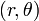$(r,\theta)$ and have the equations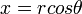$x = rcos\theta$ and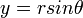$y = rsin\theta$

Hence,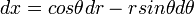$dx = cos\theta dr - rsin\theta d\theta$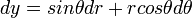$dy = sin\theta dr + rcos\theta d\theta$

Hence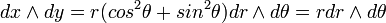$dx\wedge dy = r(cos^2\theta + sin^2\theta)dr\wedge d\theta = rdr\wedge d\theta$

Now, the planimeter is essentially a 1 form corresponding to the speed of the wheel. We consider a diagram where the angle from the horizontal at the fixed end of the planimeter to the measuring end is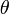$\theta$ and the angle from the horizontal to the first rod (the one connected to the fixed point) is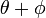$\theta + \phi$. Hence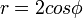$r = 2cos\phi$ and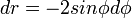$dr = -2sin\phi d\phi$

With a little plane geometry we can see that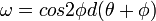$\omega = cos2\phi d(\theta + \phi)$

Computing,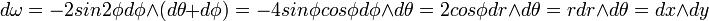$d\omega = -2 sin2\phi d\phi\wedge(d\theta + d\phi) = -4 sin\phi cos\phi d\phi\wedge d\theta = 2cos\phi dr\wedge d\theta = rdr\wedge d\theta = dx\wedge dy$

Now applying stokes theorem, the the planimeter integrates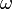$\omega$ over the boundary of our surface and hence this is just the integral of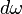$d\omega$ over the surface. But this is just the integral of the area form.

Hence the planimeter measure the area of a surface.

Back to Stokes Theorem

Firstly recall that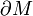$\partial M$ is oriented so that if you prepend the outward normal to its orientation you get the orientation of M

Alternatively we recall that neighborhoods of points on the boundary look like the half space. Hence we can choose to restrict our attention to atlas's where all charts look like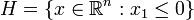$H= \{x\in\mathbb{R}^n:x_1\leq 0\}$

We can see that these orientations are the same, i.e., just prepend the outward normal to the half space.

Proof of Stokes

We have now defined all the terms. WLOG$\omega$ is supported in one chart (by linearity)

For a compactly supported n-1 form on H need to show that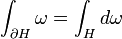$\int_{\partial H}\omega = \int_H d\omega$

We let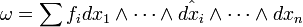$\omega = \sum f_i dx_1\wedge\cdots\wedge \hat{dx_i}\wedge\cdots\wedge dx_n$ (where the hat means it is omitted)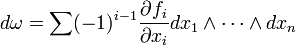$d\omega = \sum (-1)^{i-1} \frac{\partial f_i}{\partial x_i} dx_1\wedge\cdots\wedge dx_n$

So,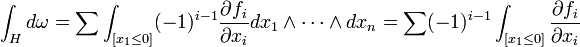$\int_H d\omega = \sum \int_{[x_1\leq 0]}(-1)^{i-1}\frac{\partial f_i}{\partial x_i}dx_1\wedge\cdots\wedge dx_n = \sum (-1)^{i-1}\int_{[x_1\leq 0]}\frac{\partial f_i}{\partial x_i}$

via fundamental theorem of calculus and that the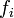$f_i$'s are compactly supported we get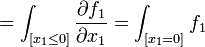$= \int_{[x_1\leq 0]} \frac{\partial f_1}{\partial x_1} = \int_{[x_1=0]} f_1$

Hence with the standard inclusion of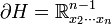$\partial H = \mathbb{R}^{n-1}_{x_2\cdots x_n}$ we get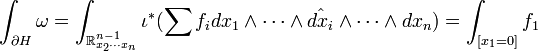$\int_{\partial H}\omega = \int_{\mathbb{R}^{n-1}_{x_2\cdots x_n}}\iota^*(\sum f_i dx_1\wedge\cdots\wedge \hat{dx_i}\wedge\cdots\wedge dx_n) = \int_{[x_1=0]}f_1$

Thus these are the same and the theorem is proved Q.E.D.

Real Plane

Consider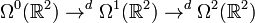$\Omega^0(\mathbb{R}^2)\rightarrow^d\Omega^1(\mathbb{R}^2)\rightarrow^d\Omega^2(\mathbb{R}^2)$

Forms in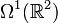$\Omega^1(\mathbb{R}^2)$ look like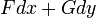$Fdx +Gdy$ and map under d to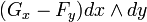$(G_x - F_y)dx\wedge dy$

Hence applying Stokes' Theorem: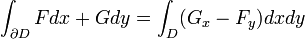$\int_{\partial D}Fdx + Gdy = \int_D (G_x-F_y)dxdy$

This is known as Greens Theorem

In complex analysis we also have a similar result Cauchy's Theorem where the integral of an analytic function around a closed path is zero. This is because analytic functions obey the Cauchy-Riemann equations and hence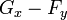$G_x-F_y$ is identically zero.

### Second Hour

Example 2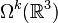$\Omega^k(\mathbb{R}^3)$

Recall previous we had consider the spaces$\Omega^k(\mathbb{R}^3)$ and showed that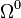$\Omega^0$ and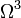$\Omega^ 3$ corresponded with functions and that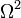$\Omega^2$ and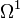$\Omega^1$ corresponded with triples of functions (i.e. vector fields). We also showed that the d function^s between these spaces are the gradient, curl and divergence functions from vector calculus.

We are now interested in integrating, using Stokes Theorem, forms in these spaces.

First, note that to a 0 manifold, assigning an orientation to the manifold is just assigning a plus or minus sign to the manifold as a result of it having a trivial basis.

This is consistent with 0 manifolds being the boundary of 1 manifolds. Indeed,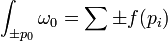$\int_{\pm p_0}\omega_0 = \sum\pm f(p_i)$

Now consider a path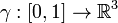$\gamma:[0,1]\rightarrow\mathbb{R}^3$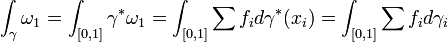$\int_{\gamma}\omega_1 = \int_{[0,1]}\gamma^*\omega_1 = \int_{[0,1]}\sum f_i d\gamma^*(x_i) = \int_{[0,1]}\sum f_i d\gamma_i$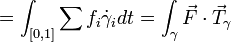$= \int_{[0,1]}\sum f_i\dot{\gamma}_i dt = \int_{\gamma}\vec F\cdot \vec T_{\gamma}$

Now lets compute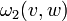$\omega_2(v,w)$

First,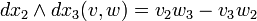$dx_2\wedge dx_3 (v,w) = v_2 w_3 - v_3 w_2$

Likewise for each component of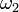$\omega_2$ we thus get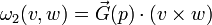$\omega_2(v,w) = \vec G(p)\cdot (v\times w)$ where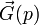$\vec G(p)$ is the vector of coefficients of$\omega_2$

Now we know that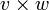$v\times w$ is a vector perpendicular to v and w with magnitude equal to the area of the defined parallelogram. So,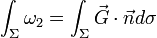$\int_{\Sigma}\omega_2 = \int_{\Sigma} \vec G\cdot \vec n d\sigma$

where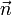$\vec n$ denotes the normal vector and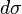$d\sigma$ is the area form and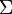$\Sigma$ is a surface

Now for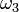$\omega_3$,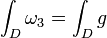$\int_D \omega_3 = \int_D g$

now,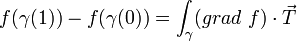$f(\gamma(1)) - f(\gamma(0)) = \int_{\gamma} (grad\ f)\cdot\vec T$

and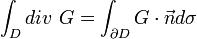$\int_D div\ G = \int_{\partial D} G\cdot\vec n d\sigma$

This is Gauss' Divergence Theorem.

We can think about this as saying that the flow from each point in a domain, when summed up, will be just the flow out of the boundary of the domain.

We also get Stokes' Theorem: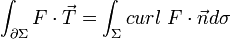$\int_{\partial\Sigma} F\cdot\vec T = \int_{\Sigma} curl\ F\cdot\vec n d\sigma$

End of Example

We recall that since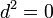$d^2 = 0$, if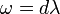$\omega = d\lambda$ then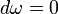$d\omega = 0$. But is the converse true? The following Lemma says 'yes', if the domain is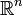$\mathbb{R}^n$

Poincare's Lemma

On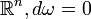$\mathbb{R}^n, d\omega = 0$ iff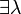$\exists\lambda$ such that$\omega = d\lambda$

This is NOT true for general M, as our homework assignment showed since we had a form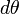$d\theta$ that had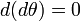$d(d\theta) = 0$ but was not d of a form.

Likewise, on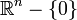$\mathbb{R}^n-\{0\}$ we have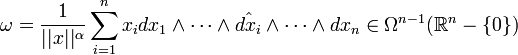$\omega = \frac{1}{||x||^{\alpha}}\sum_{i=1}^{n}x_i dx_1\wedge\cdots\wedge\hat{dx_i}\wedge\cdots\wedge dx_n \in\Omega^{n-1}(\mathbb{R}^n-\{0\})$

Claim:

For appropriate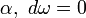$\alpha,\ d\omega = 0$ but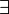$\exists$ no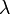$\lambda$ such that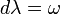$d\lambda = \omega$

This is in our next homework assignment.

Now, if there was such a$\lambda$,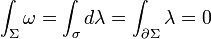$\int_{\Sigma}\omega = \int_{\sigma}d\lambda = \int_{\partial\Sigma}\lambda = 0$

If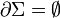$\partial\Sigma = \empty$ (such as any sphere)

But,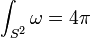$\int_{S^2}\omega = 4\pi$

Definition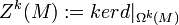$Z^k(M) := ker d|_{\Omega^k(M)}$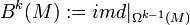$B^k(M) := im d|_{\Omega^{k-1}(M)}$

Clearly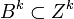$B^k\subset Z^k$ so the following definition makes sense:

Definition (de-Rham Cohomology)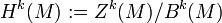$H^k(M):= Z^k(M)/B^k(M)$

Claim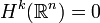$H^k(\mathbb{R}^n) = 0$ yet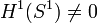$H^1(S^1)\neq 0$.

Also,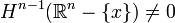$H^{n-1}(\mathbb{R}^n - \{x\})\neq 0$

### More fun with the planimeter

You can build your own low-tech planimeter out of lego!

Here my left hand is holding the fixed point steady while the right hand traces the shape. The net number of times the wheel turns while the shape is traced out indicates the area.

The empirical test: the two shapes below have the same area, and while tracing them the wheel turned almost exactly the same number of times (about 2.3 rotations). Moreover, tracing smaller shapes caused it to turn fewer times, larger shapes more.

The problem: I couldn't do better than this. Calibrating it (calculating how many rotations corresponds to exactly what area) was a nightmare. Mathematically it was possible, but my planimeter is not accurate enough to agree with my math. If you wish to build your own more accurate one, you might want to try using a thinner wheel or one that grips the table better so you don't accidently lose any turning motion.